ZHCSKJ2A November   2019  – April 2020

PRODUCTION DATA.

1. 特性
2. 应用
3. 说明
1.     Device Images
4. 修订历史记录
5. Device Comparison Table
6. Pin Configuration and Functions
7. Specifications
8. Detailed Description
1. 8.1 Overview
2. 8.2 Functional Block Diagram
3. 8.3 Feature Description
1. 8.3.1 Digital-to-Analog Converter (DAC) Architecture
2. 8.3.2 Internal Reference
3. 8.3.3 Power-On Reset (POR)
4. 8.3.4 Software Reset
4. 8.4 Device Functional Modes
5. 8.5 Programming
1. 8.5.1 Serial Interface
1. 8.5.1.1 SPI Mode
2. 8.5.1.2 I2C Mode
6. 8.6 Register Maps
1. 8.6.1 Registers
9. Application and Implementation
1. 9.1 Application Information
2. 9.2 Typical Application
3. 9.3 System Examples
4. 9.4 What To Do and What Not To Do
5. 9.5 Initialization Setup
10. 10Power Supply Recommendations
11. 11Layout
12. 12器件和文档支持
1. 12.1 文档支持
2. 12.2 相关链接
3. 12.3 接收文档更新通知
4. 12.4 支持资源
5. 12.5 商标
6. 12.6 静电放电警告
7. 12.7 Glossary
13. 13机械、封装和可订购信息

• DRX|10

### 7.10 Typical Characteristics

at TA = 25°C, VDD = 5.5 V, internal reference = 2.5 V, REF-DIV = 0 and BUFF-GAIN = 1, channel A shown, and DAC outputs unloaded (unless otherwise noted)Figure 3. Integral Linearity Error vs Digital Input Code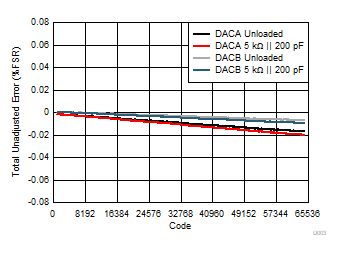Figure 5. Total Unadjusted Error vs Digital Input Code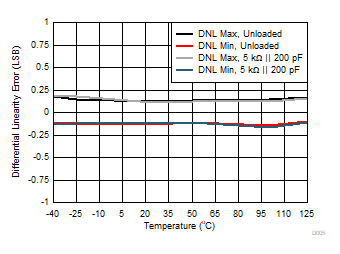Figure 7. Differential Linearity Error vs TemperatureFigure 9. Zero-Code Error vs Temperature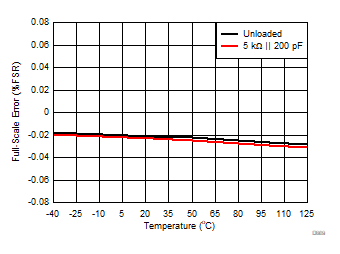Figure 11. Full-Scale Error vs TemperatureREF-DIV = 0 and BUFF-GAIN = 0
Figure 13. Integral Linearity Error vs Supply VoltageFigure 15. Total Unadjusted Error vs Supply VoltageFigure 17. Offset Error vs Supply VoltageFigure 19. Full-Scale Error vs Supply VoltageFigure 21. Differential Linearity Error vs Reference Voltage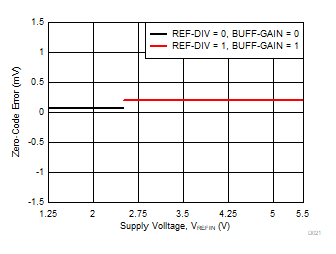Figure 23. Zero-Code Error vs Reference Voltage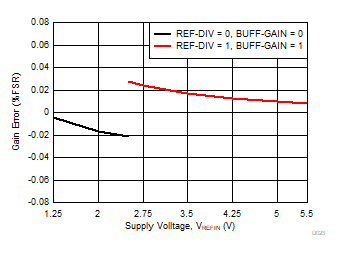Figure 25. Gain Error vs Reference VoltageFigure 27. Supply Current vs Digital Input CodeDAC code at midscale
Figure 29. Supply Current vs Supply VoltageExternal reference = 2.5 V, REF-DIV = 1 and BUFF-GAIN = 0
Figure 31. Power Down Current vs Supply VoltageREF-DIV = 0 and BUFF-GAIN = 0
Figure 33. Source and Sink Capability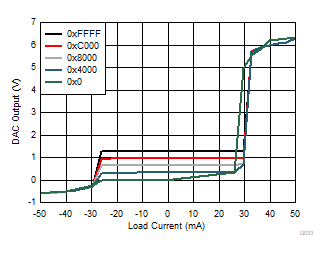REF-DIV = 1 and BUFF-GAIN = 0
Figure 35. Source and Sink Capability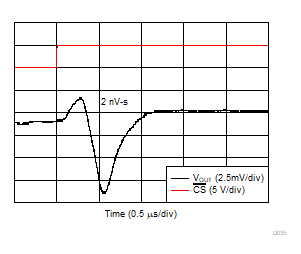DAC code transition from midscale to midscale – 1 LSB, REF-DIV = 0 and BUFF-GAIN = 0
Figure 37. Glitch Impulse, Falling Edge, 1-LSB StepREF-DIV = 0 and BUFF-GAIN = 0
Figure 39. Full-Scale Settling Time, Falling EdgeREF-DIV = 0 and BUFF-GAIN = 0
Figure 41. Power-Off Glitchfo = 1 kHz, fs = 400 kHz, includes 7 harmonics, measurement bandwidth = 20 kHz, external reference = 2.5 V, REF-DIV = 0 and BUFF-GAIN = 0
Figure 43. DAC Output THD+N vs FrequencyDAC code at midscale, external reference = 2.5 V, REF-DIV = 0 and BUFF-GAIN = 0
Figure 45. DAC Output Noise: 0.1 Hz to 10 HzSCLK = 1 MHz, DAC code at midscale, external reference = 2.5 V, REF-DIV = 0 and BUFF-GAIN = 0
Figure 47. Clock FeedthroughFigure 49. Internal Reference Voltage vs Supply VoltageFigure 51. Internal Reference Noise Density vs FrequencyFigure 53. Internal Reference Temperature Drift HistogramFigure 55. Internal Reference Temperature Drift (Pre-Solder and Post-Solder) HistogramFigure 4. Differential Linearity Error vs Digital Input CodeFigure 6. Integral Linearity Error vs Temperature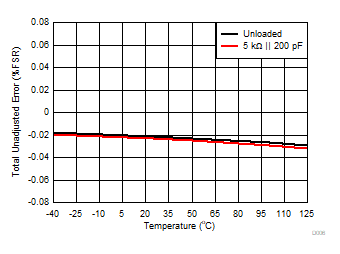Figure 8. Total Unadjusted Error vs Temperature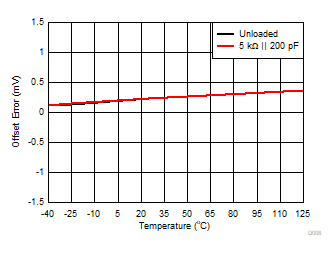Figure 10. Offset Error vs TemperatureFigure 12. Gain Error vs TemperatureREF-DIV = 0 and BUFF-GAIN = 0
Figure 14. Differential Linearity Error vs Supply VoltageFigure 16. Zero-Code Error vs Supply VoltageFigure 18. Gain Error vs Supply VoltageFigure 20. Integral Linearity Error vs Reference VoltageFigure 22. Total Unadjusted Error vs Reference VoltageFigure 24. Offset Error vs Reference Voltage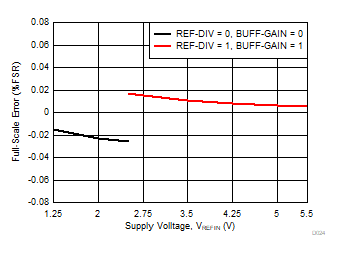Figure 26. Full-Scale Error vs Reference VoltageDAC code at midscale
Figure 28. Supply Current vs TemperatureREF-DIV = 0 and BUFF-GAIN = 0
Figure 30. Power-Down Current vs TemperatureExternal reference = 2.5 VREF-DIV = 0 and BUFF-GAIN = 1
Figure 34. Source and Sink Capability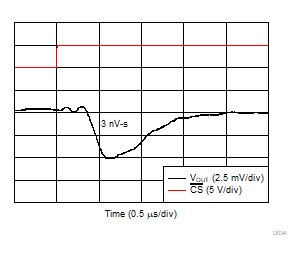DAC code transition from midscale – 1 to midscale LSB, REF-DIV = 0 and BUFF-GAIN = 0
Figure 36. Glitch Impulse, Rising Edge, 1-LSB StepREF-DIV = 0 and BUFF-GAIN = 0
Figure 38. Full-Scale Settling Time, Rising EdgeREF-DIV = 0 and BUFF-GAIN = 0
Figure 40. Power-On GlitchDAC code at midscale, VDD = 5.0 V + 0.2 VPP, REF-DIV = 0 and BUFF-GAIN = 0
Figure 42. DAC Output AC PSRR vs Frequency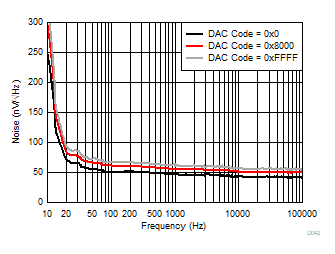Gain = 1X (REF-DIV = 1 and BUFF-GAIN = 1), external reference = 2.5 V, REF-DIV = 0 and BUFF-GAIN = 0
Figure 44. DAC Output Noise Spectral DensityDAC code at midscale, internal reference = 2.5 V, REF-DIV = 0 and BUFF-GAIN = 0
Figure 46. DAC Output Noise: 0.1 Hz to 10 Hz30 units
Figure 48. Internal Reference Voltage vs TemperatureFigure 50. Internal Reference Voltage vs TimeFigure 52. Internal Reference Noise: 0.1 Hz to 10 HzFigure 54. Internal Reference Initial Accuracy
(Pre- and Post-Solder) Histogram